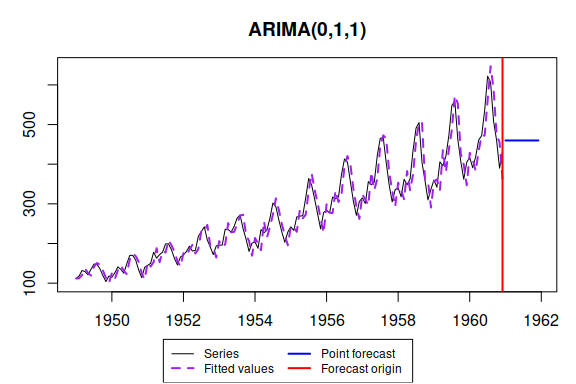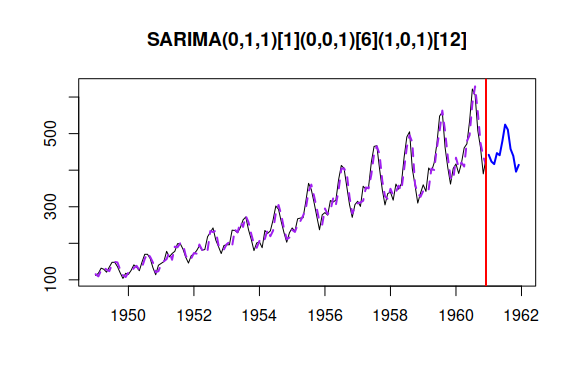# ssarima() - State-Space ARIMA

#### 2023-01-17

SSARIMA stands for “State-space ARIMA” or “Several Seasonalities ARIMA”. Both names show what happens in the heart of the function: it constructs ARIMA in a state-space form and allows to model several (actually more than several) seasonalities. ssarima() is a function included in smooth package. This vignette covers ssarima() and auto.ssarima() functions. For more details about the underlying model, read (Svetunkov and Boylan 2019).

As usual, we will use data from Mcomp package, so it is advised to install it.

require(smooth)

The default call constructs ARIMA(0,1,1):

ssarima(AirPassengers, h=12, silent=FALSE)
## Time elapsed: 0.01 seconds
## Model estimated: ARIMA(0,1,1)
## Matrix of MA terms:
##        Lag 1
## MA(1) 0.4066
## Initial values were produced using backcasting.
##
## Loss function type: likelihood; Loss function value: 700.9086
## Error standard deviation: 31.6734
## Sample size: 144
## Number of estimated parameters: 2
## Number of degrees of freedom: 142
## Information criteria:
##      AIC     AICc      BIC     BICc
## 1405.817 1405.902 1411.757 1411.968Some more complicated model can be defined using parameter orders the following way:

ssarima(AirPassengers, orders=list(ar=c(0,1),i=c(1,0),ma=c(1,1)), lags=c(1,12), h=12)
## Time elapsed: 0.05 seconds
## Model estimated: SARIMA(0,1,1)(1,0,1)
## Matrix of AR terms:
##       Lag 12
## AR(1)      1
## Matrix of MA terms:
##         Lag 1 Lag 12
## MA(1) -0.3269 0.1017
## Initial values were produced using backcasting.
##
## Loss function type: likelihood; Loss function value: 554.9947
## Error standard deviation: 11.58
## Sample size: 144
## Number of estimated parameters: 4
## Number of degrees of freedom: 140
## Information criteria:
##      AIC     AICc      BIC     BICc
## 1117.989 1118.277 1129.869 1130.584

This would construct seasonal ARIMA(0,1,1)(1,0,1)$$_{12}$$.

We could try selecting orders manually, but this can also be done automatically via auto.ssarima() function:

auto.ssarima(AirPassengers, h=12)
## Time elapsed: 2.47 seconds
## Model estimated: SARIMA(0,1,3)(0,1,0)
## Matrix of MA terms:
##         Lag 1
## MA(1) -0.3587
## MA(2)  0.1056
## MA(3) -0.2230
## Initial values were produced using backcasting.
##
## Loss function type: likelihood; Loss function value: 549.9204
## Error standard deviation: 11.179
## Sample size: 144
## Number of estimated parameters: 4
## Number of degrees of freedom: 140
## Information criteria:
##      AIC     AICc      BIC     BICc
## 1107.841 1108.129 1119.720 1120.435

Automatic order selection in SSARIMA with optimised initials does not work well and in general is not recommended. This is partially because of the possible high number of parameters in some models and partially because of potential overfitting of first observations when non-zero order of AR is selected:

auto.ssarima(AirPassengers, h=12, initial="backcasting")
## Time elapsed: 2.46 seconds
## Model estimated: SARIMA(0,1,3)(0,1,0)
## Matrix of MA terms:
##         Lag 1
## MA(1) -0.3587
## MA(2)  0.1056
## MA(3) -0.2230
## Initial values were produced using backcasting.
##
## Loss function type: likelihood; Loss function value: 549.9204
## Error standard deviation: 11.179
## Sample size: 144
## Number of estimated parameters: 4
## Number of degrees of freedom: 140
## Information criteria:
##      AIC     AICc      BIC     BICc
## 1107.841 1108.129 1119.720 1120.435
auto.ssarima(AirPassengers, h=12, initial="optimal")
## Time elapsed: 23.14 seconds
## Model estimated: SARIMA(0,1,3)(0,1,0) with drift
## Matrix of MA terms:
##         Lag 1
## MA(1) -0.3645
## MA(2)  0.1120
## MA(3) -0.2297
## Constant value is: 0.2186
## Initial values were optimised.
##
## Loss function type: likelihood; Loss function value: 549.269
## Error standard deviation: 11.7305
## Sample size: 144
## Number of estimated parameters: 18
## Number of degrees of freedom: 126
## Information criteria:
##      AIC     AICc      BIC     BICc
## 1134.538 1140.010 1187.995 1201.592

As can be seen from the example above the model with optimal initials takes more time and we end up with a different model than in the case of backcasting.

A power of ssarima() function is that it can estimate SARIMA models with multiple seasonalities. For example, SARIMA(0,1,1)(0,0,1)_6(1,0,1)_12 model can be estimated the following way:

ssarima(AirPassengers, orders=list(ar=c(0,0,1),i=c(1,0,0),ma=c(1,1,1)), lags=c(1,6,12), h=12, silent=FALSE)

It probably does not make much sense for this type of data, it would make more sense on high frequency data (for example, taylor series from forecast package). However, keep in mind that multiple seasonal ARIMAs are very slow in estimation and are very capricious. So it is really hard to obtain an appropriate and efficient multiple seasonal ARIMA model. To tackle this issue, I’ve developed an alternative ARIMA model for multiple seasonalities, called msarima().

Now let’s introduce some artificial exogenous variables:

x <- cbind(rnorm(length(AirPassengers),50,3),rnorm(length(AirPassengers),100,7))

If we save model:

ourModel <- auto.ssarima(AirPassengers, h=12, holdout=TRUE, xreg=x)

we can then reuse it:

ssarima(AirPassengers, model=ourModel, h=12, holdout=FALSE, xreg=x, interval=TRUE)
## Time elapsed: 0.24 seconds
## Model estimated: SARIMAX(0,1,3)(0,1,0) with drift
## Matrix of MA terms:
##         Lag 1
## MA(1) -0.2492
## MA(2)  0.1179
## MA(3) -0.2280
## Constant value is: 0.37
## Initial values were provided by user.
## Xreg coefficients were estimated in a normal style
##
## Loss function type: likelihood; Loss function value: 550.9983
## Error standard deviation: 11.1442
## Sample size: 144
## Number of estimated parameters: 1
## Number of provided parameters: 19
## Number of degrees of freedom: 143
## Information criteria:
##      AIC     AICc      BIC     BICc
## 1103.997 1104.025 1106.966 1107.036
##
## 95% parametric prediction interval was constructed

Finally, we can combine several SARIMA models:

ssarima(AirPassengers, h=12, holdout=FALSE, interval=TRUE, combine=TRUE)
## Time elapsed: 0.01 seconds
## Model estimated: ARIMA(0,1,1)
## Matrix of MA terms:
##        Lag 1
## MA(1) 0.4066
## Initial values were produced using backcasting.
##
## Loss function type: likelihood; Loss function value: 700.9086
## Error standard deviation: 31.6734
## Sample size: 144
## Number of estimated parameters: 2
## Number of degrees of freedom: 142
## Information criteria:
##      AIC     AICc      BIC     BICc
## 1405.817 1405.902 1411.757 1411.968
##
## 95% parametric prediction interval was constructed

# MSARIMA

While SSARIMA is flexible, it is not fast. In fact, it cannot handle high frequency data well and most probably will take ages to estimate the parameter and produce forecasts. This is because of the transition matrix, which becomes huge in case of multiple seasonalities. The MSARIMA model (Multiple Seasonal ARIMA) is formulated in a different state-space form, which reduces the size of transition matrix, significantly reducing the computational time for cases with high frequency data.

There are auto.msarima() and msarima() function in the package, that do things similar to auto.ssarima() and ssarima(). Here’s just one example of what can be done with it:

msarima(AirPassengers, orders=list(ar=c(0,0,1),i=c(1,0,0),ma=c(1,1,1)),lags=c(1,6,12),h=12, silent=FALSE)
## Time elapsed: 0.27 seconds
## Model estimated using msarima() function: SARIMA(0,1,1)(0,0,1)(1,0,1)
## Distribution assumed in the model: Normal
## Loss function type: likelihood; Loss function value: 633.0421
## ARMA parameters of the model:
## AR:
## phi1
##   0.2527
## MA:
##  theta1  theta1 theta1
##     0.1616     0.1505     0.5045
##
## Sample size: 144
## Number of estimated parameters: 24
## Number of degrees of freedom: 120
## Information criteria:
##      AIC     AICc      BIC     BICc
## 1314.084 1324.168 1385.360 1410.418The forecasts of the two models might differ due to the different state space form. The detailed explanation of MSARIMA is given in Chapter 9 of ADAM textbook.